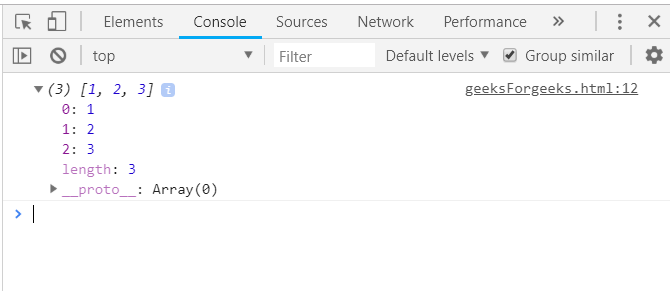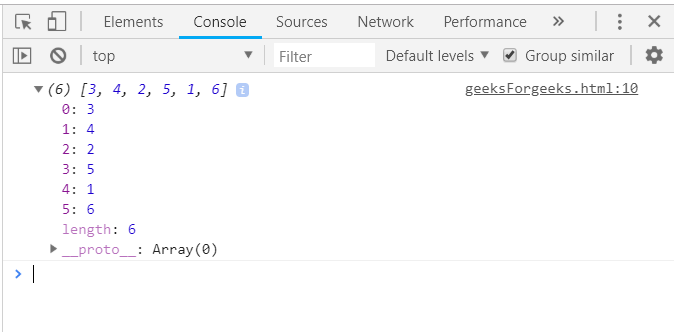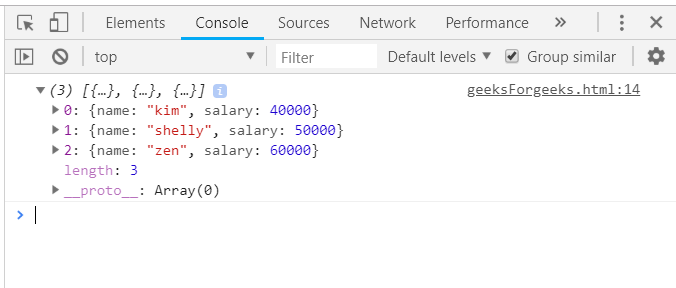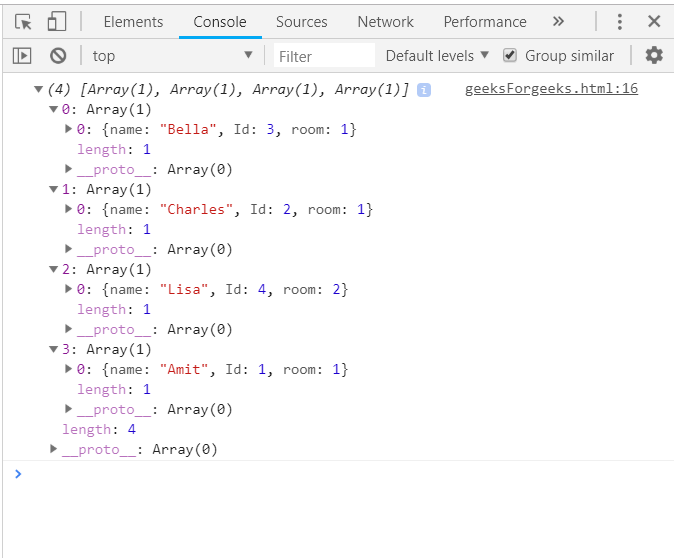Open in App
Not now

# Underscore.js _.sortBy Function

• Last Updated : 26 Oct, 2022

The Underscore.js is a JavaScript library that provides a lot of useful functions that helps in the programming in a big way like the map, filter, invoke etc even without using any built-in objects. The _.sortBy() function is used to sort all the elements of the list in ascending order according to the function given to it as a parameter. Passing the array with a function which returns the number and it will sort the array in ascending order and return an array. The array can be both of numeric values and string values. Syntax:

`_.sortBy(list, iterate, [context]) `

Parameters: This function accepts three parameters as mentioned above and described below:

• List: This parameter is used to set the list of items.
• Iterate: This parameter is used to hold the test condition.
• Context: This parameter is used to display the content.

Return values: It returns a sorted array which is being sorted according to the function passed. Using a function which simply returns a number: The ._sortBy() function takes the element from the list one by one and do the specified operations given in the function. Like here the function is just to sort all the elements of the list. After traversing and sorting all the elements, the sortBy() function ends. Now, the variable which stores the original array will contain the sorted array. Example:

## html

 `<``html``>``    ``<``head``>``        ``<``script` `type="text/javascript" src=``        ``"https://cdnjs.cloudflare.com/ajax/libs/underscore.js/1.9.1/underscore.js">``        ````    ````    ``<``body``>``        ``<``script` `type="text/javascript">``            ``var array = _.sortBy([2, 3, 1], function(num) {``            ``return num;``            ``});``            ``console.log(array);``        ````    ````                   `

Output:Using Math.cos() function: Passing a list of numbers and do operations like taking ‘cos()’ of the numbers and then comparing them to sort the array. In the same manner apply all the “math’ function like tan(), sin(), cot() etc to sort the array. Example:

## html

 `<``html``>``    ``<``head``>``        ``<``script` `type="text/javascript" src=``        ``"https://cdnjs.cloudflare.com/ajax/libs/underscore.js/1.9.1/underscore.js">``        ````    ````    ``<``body``>``        ``<``script` `type="text/javascript">``            ``console.log(_.sortBy([1, 2, 3, 4, 5, 6], function(num) {``                ``return Math.cos(num);``            ``}));   ``        ````    ````                   `

Output:Using a property of the array: Apply _.sortBy() method to strings and first declare the array (here array is ‘arr’). Choose one property of the array on the basis of which need to sort like here ‘name’. Console.log the sorted array by passing the array and that property. Example:

## html

 `<``html``>``    ``<``head``>``        ``<``script` `type="text/javascript" src=``        ``"https://cdnjs.cloudflare.com/ajax/libs/underscore.js/1.9.1/underscore.js">``        ````    ````    ``<``body``>``        ``<``script` `type="text/javascript">``            ``var arr = [``                 ``{name: 'kim', salary: 40000},``                 ``{name: 'shelly', salary: 50000},``                 ``{name: 'zen', salary: 60000}``            ``];``            ``console.log(_.sortBy(arr, 'name'));``        ````    ````                   `

Output:Reversing an array using _.sortBy() function: Although, the _.sortBy() function sorts in an ascending order but still reverse the array with the help of yet another function ‘reverse()’ along with _.sortBy(). First, _.sortBy() function will sort the list in ascending order and then the ‘reverse()’ function will make the array reversed. Finally, print the array. Example:

## html

 `<``html``>``    ``<``head``>``        ``<``script` `type="text/javascript" src=``        ``"https://cdnjs.cloudflare.com/ajax/libs/underscore.js/1.9.1/underscore.js">``        ````    ````    ``<``body``>``        ``<``script` `type="text/javascript">``            ``var patients = [``                                 ``[{name: 'Amit', Id: 1, room: 1}],``                                 ``[{name: 'Lisa', Id: 4, room: 2}],``                                 ``[{name: 'Charles', Id: 2, room: 1}],``                                 ``[{name: 'Bella', Id: 3, room: 1}]``                               ``];``            ``var descending = _.sortBy(patients, 'total').reverse();``            ``console.log(descending);``        ````    ````                   `

Output:jQuery is an open source JavaScript library that simplifies the interactions between an HTML/CSS document, It is widely famous with it’s philosophy of “Write less, do more”. You can learn jQuery from the ground up by following this jQuery Tutorial and jQuery Examples.

My Personal Notes arrow_drop_up